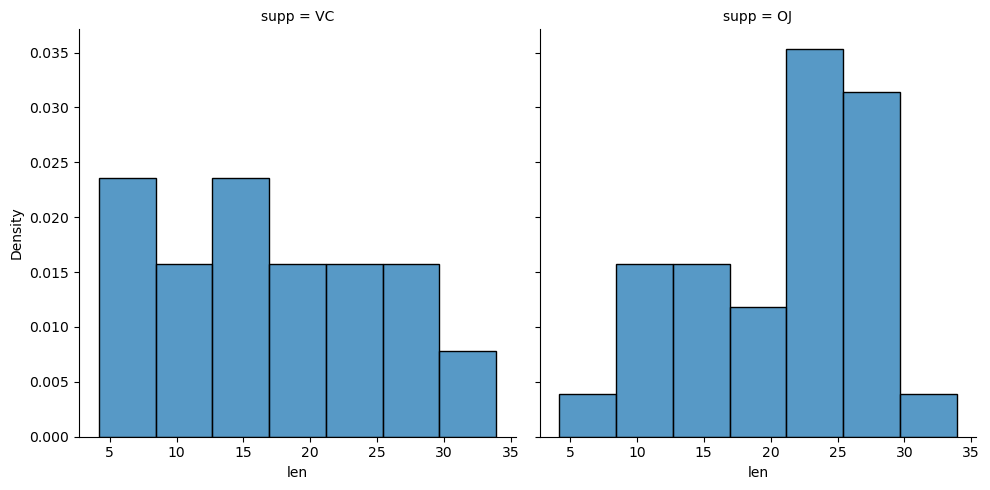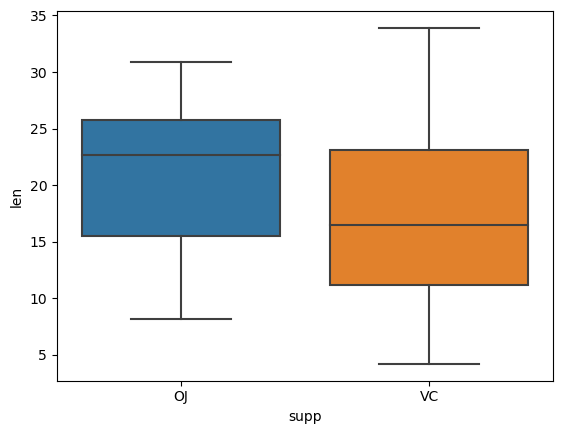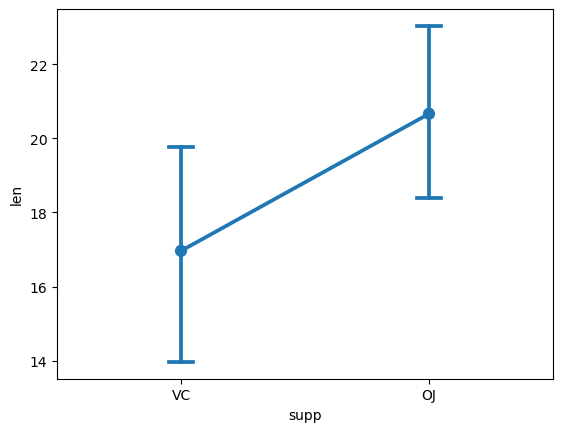# How to create bivariate plots to compare groups

## Description

Suppose we have a dataset with different treatment conditions and an outcome variable, and we want to perform exploratory data analysis. How would we visually compare the treatment conditions with regards to the outcome variable?

## Using Matplotlib and Seaborn, in Python

View this solution alone.

The solution below uses an example dataset about the teeth of 10 guinea pigs at three Vitamin C dosage levels (in mg) with two delivery methods (orange juice vs. ascorbic acid). (See how to quickly load some sample data.)

1
2
from rdatasets import data
df = data('ToothGrowth')


If you wish to understand the distribution of a numeric variable (here “len”) compared across different values of a categorical variable (here “supp”), you can construct a bivariate histogram. We use Seaborn and Matplotlib to do so.

1
2
3
4
import seaborn as sns
import matplotlib.pyplot as plt
sns.displot(df, x="len", col="supp", stat="density")
plt.show()To visualize the same information summarized using quartiles only, you can construct a bivariate box plot.

1
2
sns.boxplot(x="supp", y="len", data = df, order = ['OJ','VC'])
plt.show()Even more simply, we may wish to plot just the means and 95% confidence intervals around the mean for the quantitative variable, for each of the values of the categorical variable. We do so with a point plot.

1
2
3
4
sns.pointplot(x = 'supp', y = 'len', data = df,
ci = 95,        # Which confidence interval?  Here 95%.
capsize = 0.1)  # Size of "cap" drawn on each confidence interval.
plt.show()

1
2
3
4
5
/tmp/ipykernel_6175/1597037981.py:1: FutureWarning:

The ci parameter is deprecated. Use errorbar=('ci', 95) for the same effect.

sns.pointplot(x = 'supp', y = 'len', data = df,See a problem? Tell us or edit the source.

## Using lattice and gplots, in R

View this solution alone.

We use a built-in dataset called ToothGrowth that discusses the length of the teeth (len) in each of 10 guinea pigs at three Vitamin C dosage levels ($0.5$, $1$, and $2$ mg) with two delivery methods - orange juice or ascorbic acid (supp).

1
2
# You can replace this example data frame with your own data
df <- ToothGrowth


If you wish to understand the distribution of the length of the tooth based on the delivery methods, you can construct a bivariate histogram plot.

1
2
3
# install.packages( "lattice" ) # if you have not already done this
library(lattice)
histogram( ~ len | supp, data = df)


To visualize the summary statistics of the length of the tooth based on the delivery methods, you can construct a bivariate box plot.

1
2
3
bwplot(df$len ~ df$supp)
# Or the following code produces a similar figure, using the mosaic package:
# boxplot(len ~ supp, data = df)


To plot the means for both treatment levels of supp for the len column, we load the gplots package and use the plotmeans function.

1
2
3
# install.packages( "gplots" ) # if you have not already done this
library(gplots)
plotmeans(df$len ~ df$supp)

1
2
3
4
5
6
Attaching package: ‘gplots’

The following object is masked from ‘package:stats’:

lowess